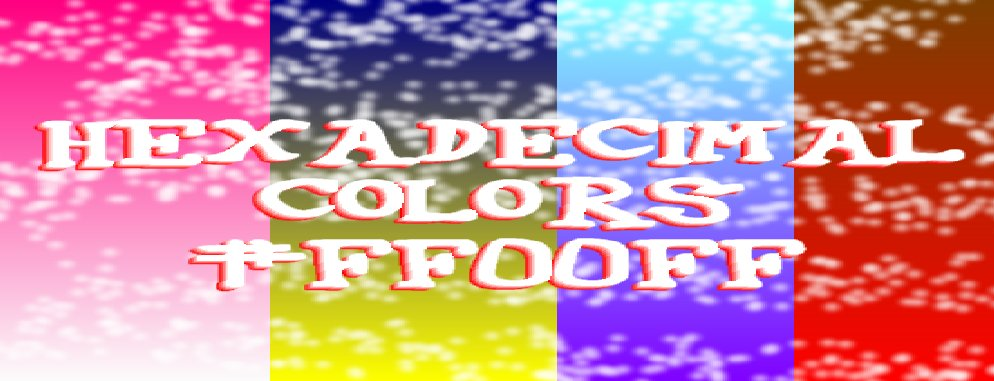# What is a hexadecimal color?

By Blaiz Enterprises  8th November 2023A hexadecimal color is a way to specify, in compact form, the red, green and blue elements (or levels) of a 24bit color (16.7 million colors) range.  Each of the red, green and blue elements of this 24bit color have a range of 0 to 255 (or 8bits) each.  They are referenced by two alpha-numerical characters, each in the range (0 to 9) and (A to F).  Each hex character yields a value of 0 to 15 (or 4bits).  The first hex character yields a large, more significant whole number value, while the second hex character yields a smaller whole number value, as shown below:

All possible 1st hex characters and their corresponding whole number values:
0 = 0
1 = 16
2 = 32
3 = 48
4 = 64
5 = 80
6 = 96
7 = 112
8 = 128
9 = 144
A = 160
B = 176
C = 192
D = 208
E = 224
F = 240

All possible 2nd hex characters and their corresponding whole number values:
0 = 0
1 = 1
2 = 2
3 = 3
4 = 4
5 = 5
6 = 6
7 = 7
8 = 8
9 = 9
A = 10
B = 11
C = 12
D = 13
E = 14
F = 15

A 24bit color is generated by mathematically adding the two whole numbers (1st and 2nd hex characters) together, to form a complete 8bit whole number value in the range of 0 to 255.  Therefore, "A3" becomes 160 + 3 = 163, same as the whole number 163.  This is done for each of the red, green and blue color elements in turn.  Red is first, then green and then blue.  The complete color equates to 3 x 8bits = 24bits in total.

A sample hexadecimal color of "A3E9DF" is calculated as follows:

Red hex value is "A3"
=> A (which is 160) + 3 = 163

Green hex value is "E9"
=> E (which is 224) + 9 = 233

Blue hex value is "DF"
=> D (which is 208) + F (which is 15) = 223

The above hexadecimal color translates into the equivalent whole number color elements of 163 (red), 233 (green), 223 (blue).

There are other, less compact, ways to define a 24bit color (non-hexadecimal color).  One is to specify the red, green and blue elements as whole numbers of say, "255, 0, 170", which in hexadecimal is #FF00AA (red/green/blue).

Notice how the 24bit hexadecimal color uses exactly 7 characters, never more, and never less.  Whereas the numerical format could use as little as "0,0,0", or 5 characters (without spaces) for black (no red, no green and no blue).  But white of "255,255,255" uses a total of 11 characters (full red, full green and full blue).

Important: